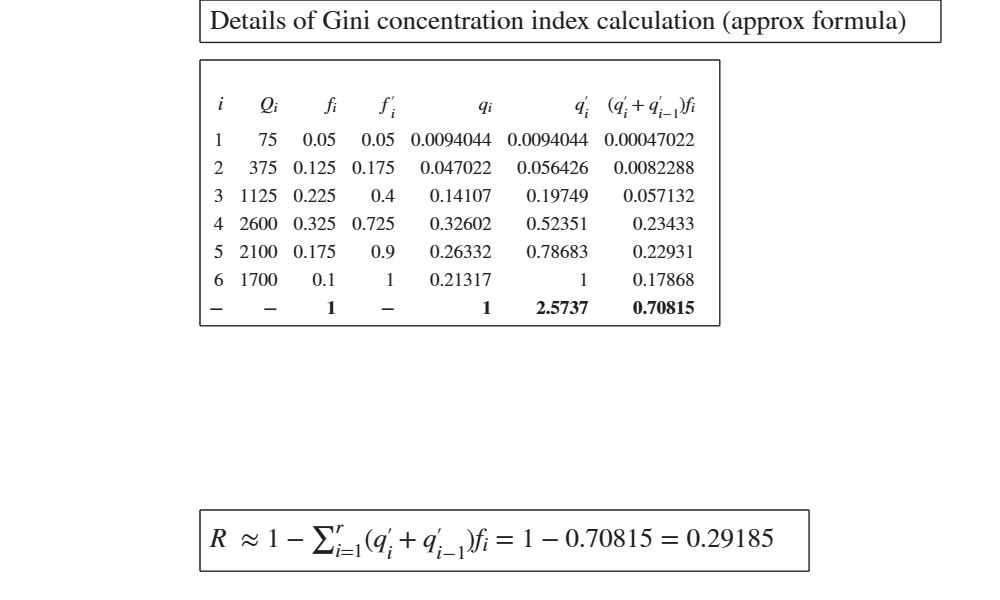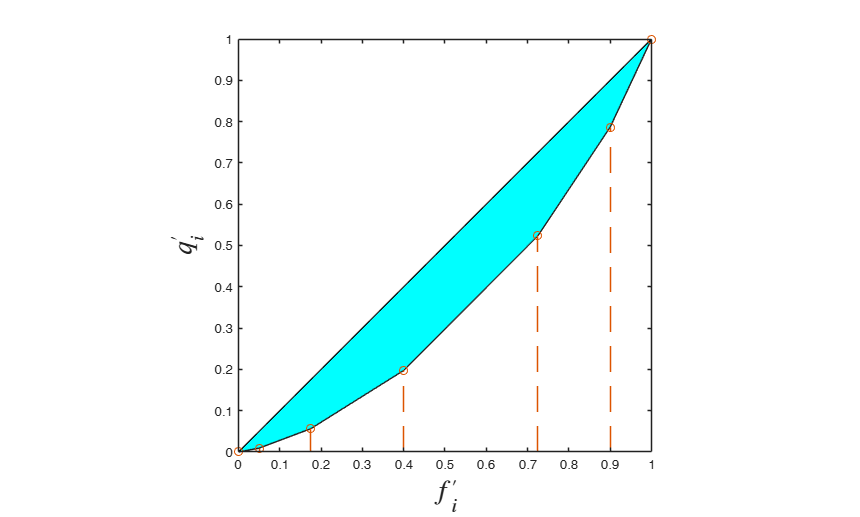GUIconcentration

GUIconcentration shows the necessary calculations to obtain the GINI concentration index in a GUI.

Syntax

• out=GUIconcentration(Q)example
• out=GUIconcentration(Q,Name,Value)example

Description

out =GUIconcentration(Q) Concentration index using exact formula.

out =GUIconcentration(Q, Name, Value) Concentrartion index using approximate formula.

Examples

expand all

Concentration index using exact formula.

The percentage breakdown of the investments of a mutual is the following.

% This is exercise 1.29 from [CMR] - page 61.
% Government bonds 55 per cent.
% Bonds 12 per cent.
% Italian equities 23 per cent
% Foreign equities 6 per cent.
% Cash 4 per cent.
% Compute concentration ratio and show the concentration area.
Q=[55 12 23 6 4];
Q=sort(Q);
out=GUIconcentration(Q,'plots',1);Concentrartion index using approximate formula.

The families in a certain area have been classified according to their annual income.

% This is exercise 1.28 from [CMR] - page 59.
% Classes of income and frequencies.
% 5  - 10	10
% 10 - 20	25
% 20 - 30	45
% 30 - 50	65
% 50 - 70	35
% 70 - 100	20
% Compute the concentration ratio and show the concentration area.
% Note that given that n is large we can use the approximate formula.
% Using as x_i the central value of the classes, we estimate the Q_i as
% x_i * n_i. For example Q_1 = 7.5 * 10, Q_2 = 15 * 25...Q_6 = 85 * 20
Q=[75 375 1125 2600 2100 1700];
ni=[10 25 45 65 35 20];
out=GUIconcentration(Q,'freq',ni,'plots',1);Input Arguments

Q — vector of non negative numeric data representing quantities. Vector.

Vector containing strictly numerical data

Data Types: double

Name-Value Pair Arguments

Specify optional comma-separated pairs of Name,Value arguments. Name is the argument name and Value is the corresponding value. Name must appear inside single quotes (' '). You can specify several name and value pair arguments in any order as Name1,Value1,...,NameN,ValueN.

Example: 'freq',1:10 , 'ExactFormula',true , 'plots',true

freq —frequencies.vector.

Vector of the same legth of Q containing the frequencies associated to each value of x. If freq is not it is assumed that all observations have the same (relative) frequency.

Example: 'freq',1:10

Data Types: double

ExactFormula —use of exact formula.boolean.

Use of exact or approximate formula.

The default is to use the exact formula if previously option freq has not been supplied, else approximate formula is used. Option ExactFormula overwrites the default.

Example: 'ExactFormula',true

Data Types: boolean

plots —show Lorenz curve.boolean.

If plots is true an additional plot which shows the concentration area is displayed on the screen. The default value of plots is false.

Example: 'plots',true

Data Types: boolean

Output Arguments

out —detailed output to compute the index. Table

Table with n+1 rows (where n is the length of x) containing what is shown in the GUI. Last row contains the totals.

Cerioli, A., Milioli, M.A., Riani, M. (2016), "Esercizi di statistica (Quinta edizione)". [CMR]